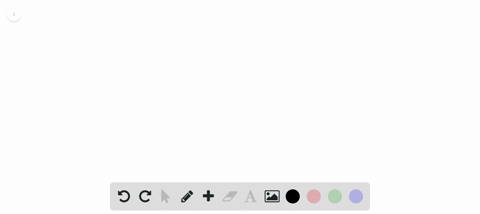Enroll in one of our FREE online STEM summer camps. Space is limited so join now!View Summer Courses### A laser beam shines along the surface of a block …

01:20Wesleyan University

Need more help? Fill out this quick form to get professional live tutoring.

Get live tutoring
Problem 7

A parallel beam of light in air makes an angle of 47.5$^\circ$ with the surface of a glass plate having a refractive index of 1.66. (a) What is the angle between the reflected part of the beam and the surface of the glass? (b) What is the angle between the refracted beam and the surface of the glass?

a) $$47.5^{\circ}$$
b) $$24.0^{\circ}$$

## Discussion

You must be signed in to discuss.

## Video Transcript

in this problem, we are asked to find the following angle. So we have a interface with air in a glass. So this is a light that strikes this place and then bounces back and some off that is refracted here. So we call this angle. This is angle of incident. Um and this is Anglo for a fraction. So that our and here we have there, Toby, they don't be reflection. So we are asked to find this angle on that. Then we are asked to far in this angles of the angle between the glass and surface off air. So those angles so it. But maybe it has to find this so we can find this angle by finding the angle of refraction which is actually equal to angle off incident. So the angle off incident we have already that is given. Then we can just subtract a 90 a minus Anglo for incident which is equal to the angle of refraction. So here we have angle off, eh? Is equal to the angle of refraction. An angle is given so we can just plug this value here in subject. Then we can get the angle between glass and reflect beam, which is 47.5. What, seven or five degrees? Then in part A. We can find that to be here. And then from there we can just subtract from 90. Tend to be and then we can find this angle. So, using a Snell's law and a done sign there angle that a is equal to physical tow N B. So I'm signed Refractive Index and be time sign. There'd be, um then we console for that. I'd be here from the relation by parking go values in N and B than the value here we get here is B is 24 degrees very 40 degrees. Then, as we did previously here by subtracting 24 from 90 Um, so then we get this angle. So 90 minus 24 that gives a 66 degrees. So this angle here is 66 degrees, and this angle here is 47.5 degrees. End off the problem. Thank you for watching